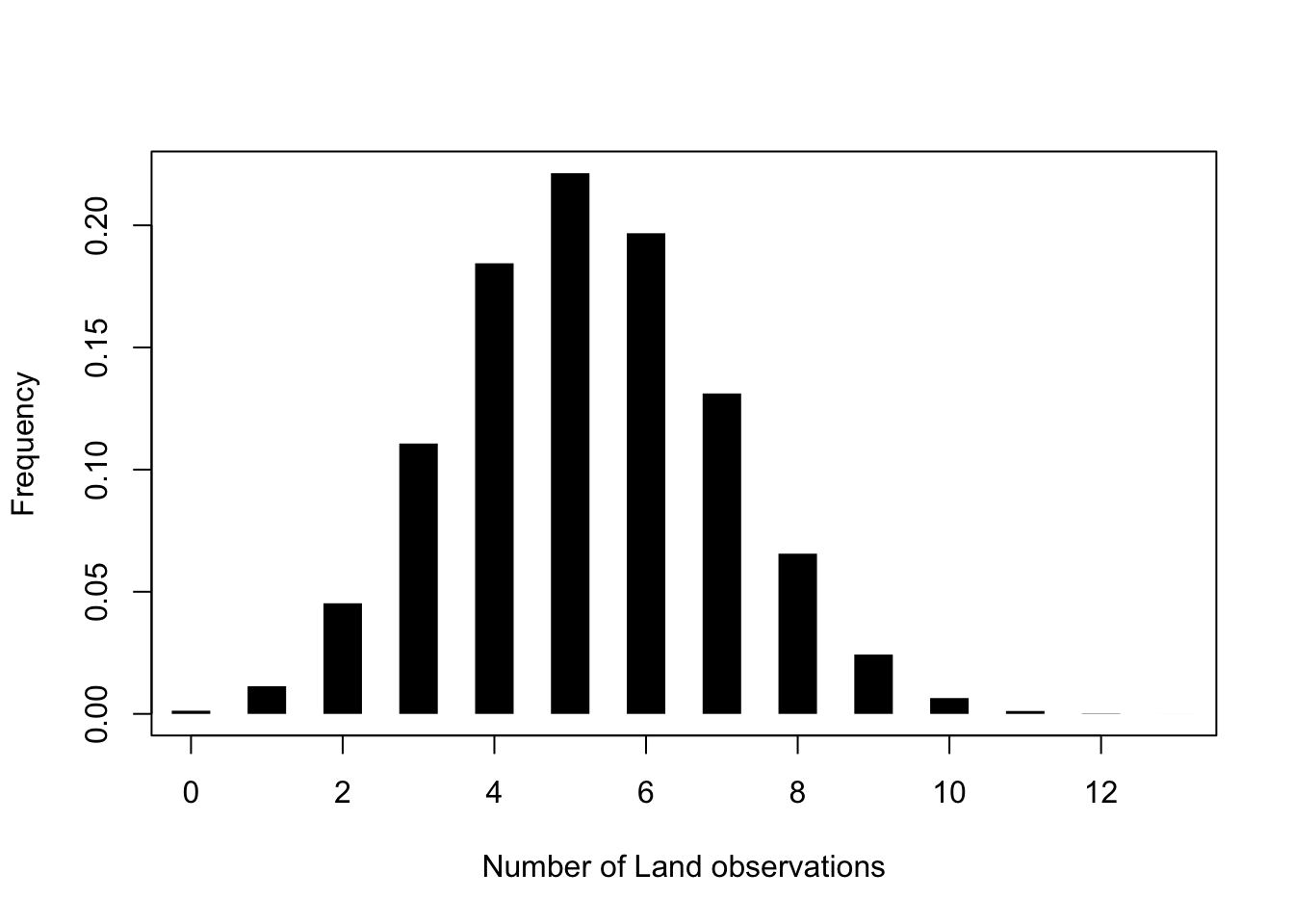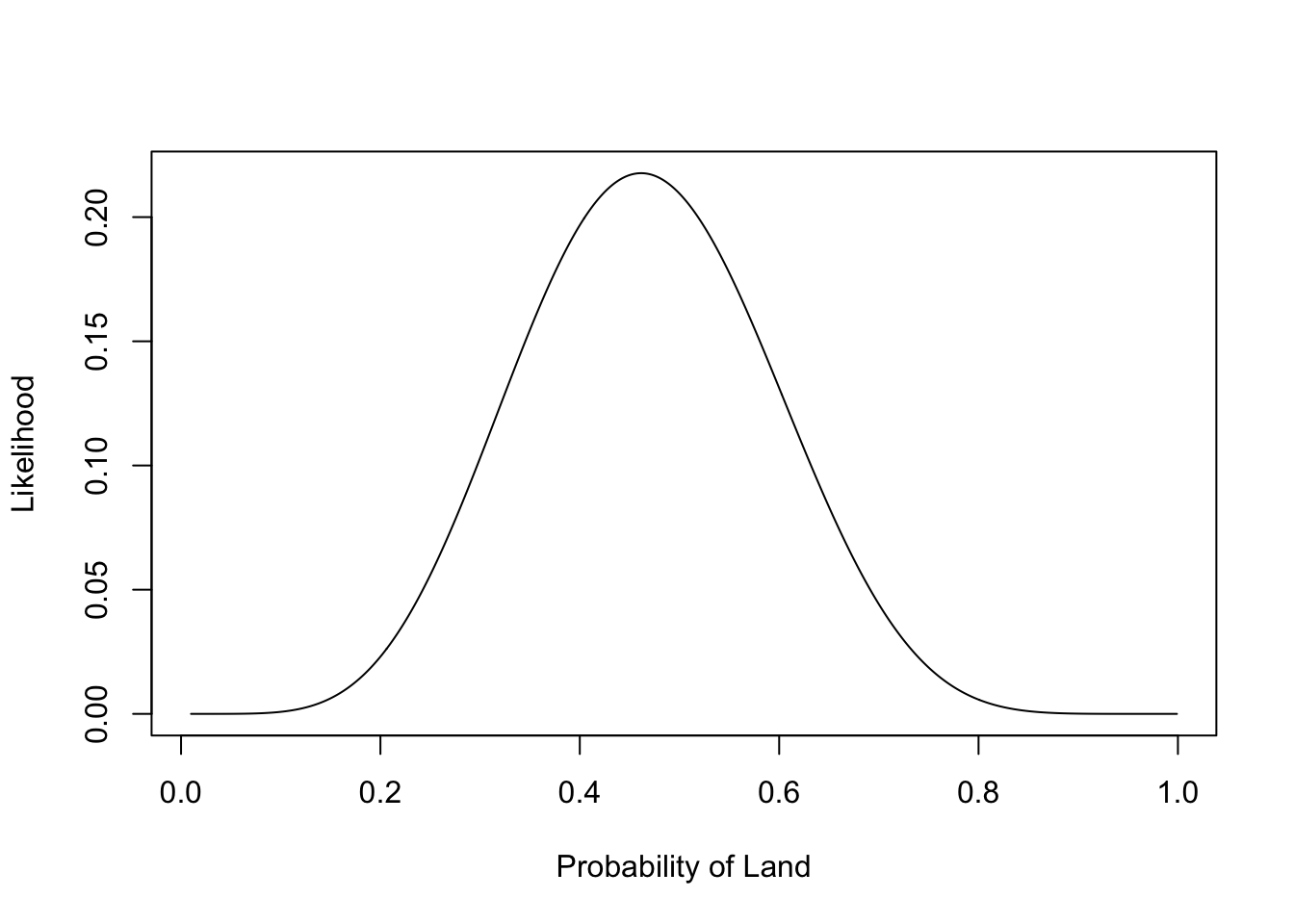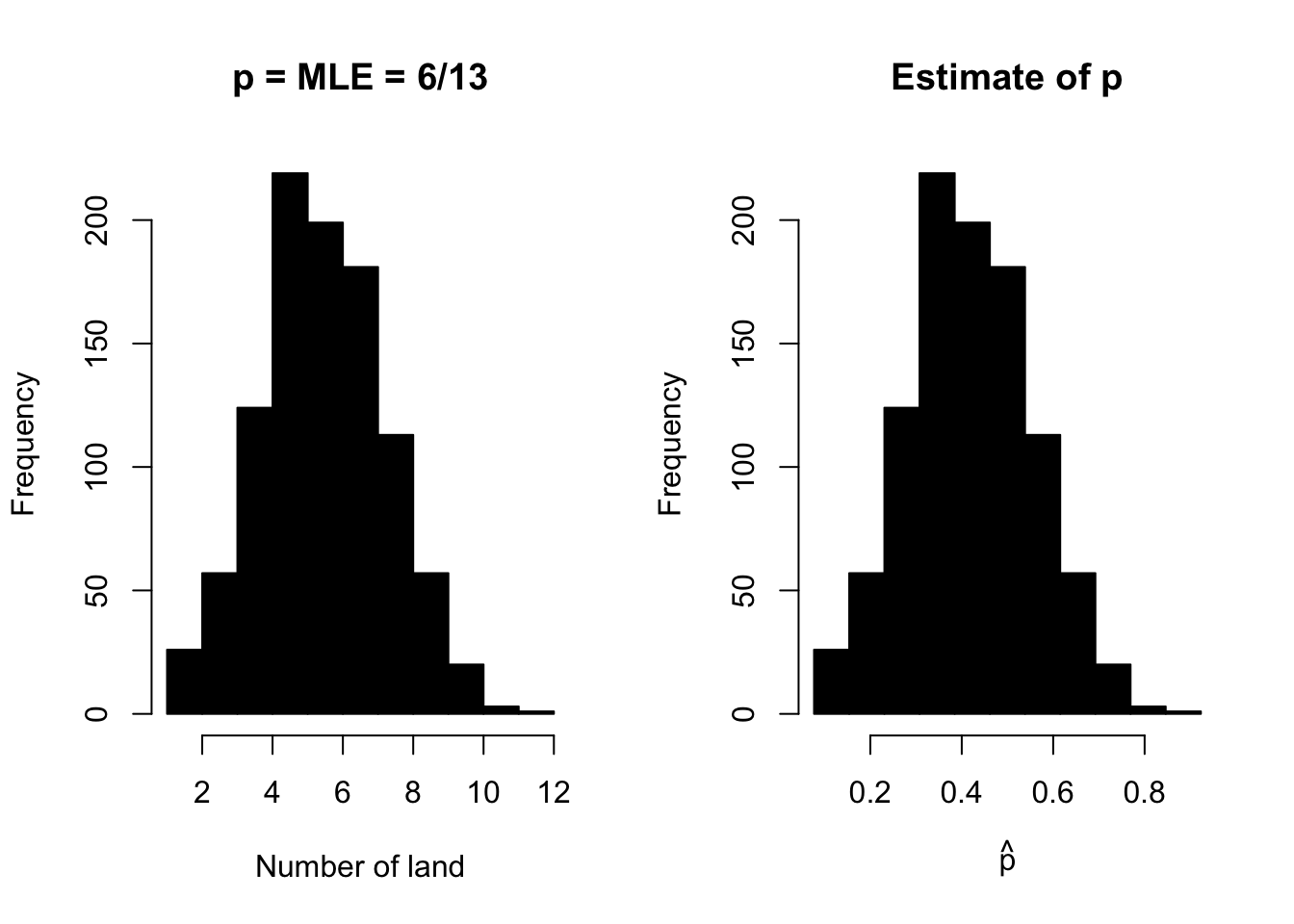# The Problem (from last week)

First, if you dare you can watch this video:

We have a globe. We have sampled 13 points (by spinning the globe around): 6 of these were land and 7 were sea. We want to know what proportion of the globe is land. We call each data point a “trial”.

Because of the way we sampled, we can assume that the probability of each point being Land is constant, and these are independent of each other. From this, we can assume that if we did the same experiment many times, the outcomes would follow a binomial distribution. Figure 1 shows a binomial distribution, i.e. the possible outcomes if we had 13 trials, and the probability of land was 0.4.

$Pr(n = r | N, p) = \frac{N !}{r! (N-r)!} p^r (1-p)^{N-r}$

PrLand <- 0.4
nTrials <- 13
n <- 0:nTrials
Pr <- dbinom(n, nTrials, PrLand)

plot(n, Pr, type="h", lwd=20, lend=3,
xlab="Number of Land observations", ylab="Frequency")Fig. 1: A binomial probability

Fig. 1 is a plot of the probabilities of different outcomes if $$p=0.4$$. But in reality we do not know what $$p$$ is: this is what we want to estimate. If we have some data (e.g. $$n=6$$), we can draw a curve that shows the probability as a function of $$p$$. We have drawn one in Fig. 2. From this we can see that we are more likely to get the data if the probability is close to 0.5, and that values from around 0.3 and 0.6 are also reasonable. But we want to be more precise about this, so we want to (a) find the value that is most likely to give the data, and (b) find some measure of spread around that which suggests what values are likely.

nLand <- 6
p <- seq(0.01, 0.999, length=500)
Pr <- dbinom(nLand, nTrials, p)

plot(p, Pr, type="l",
xlab="Probability of Land", ylab="Likelihood")Fig. 2: A binomial likelihood

## Finding the MLE

The probability, $$Pr(n = r | N, p)$$, is a mathematical description of how the data came about: it is a statistical model. We assume that there is some unknown “true” value of the parameter, and this is what we are trying to estimate. Thus the parameter is fixed, and the data are what varies. We thus want to find the parameter which is most likely to give rise to the data.

We can vary $$p$$, and calculate $$Pr(n = r | N, p)$$, so that $$Pr(n = r | N, p)$$ is a function of $$p$$. When the probability of the data is a function of the parameters, we call this the likelihood. Our aim is to find the best estimate of $$p$$, i.e. the one which has the highest likelihood of giving the data. We thus want to maximise the likelihood: the estimate we get is called the maximum likelihood estimator, which is often abbreviated to m.l.e., or MLE. There are several ways to find the mle. Here we will find an analytic solution, i.e. use pencil and paper, to calculate the expression for the m.l.e.

## Maximising the likelihood: the maths

The maximum of the likelihood is at the same value of $$p$$ as the maximum of the log-likelihood, and it is easier to woth on the log scale. So we will work with $$\log(L(p|N, r)) = l(p|N, r)$$

$l(p | n) = \log(N !) - \log(r!) - \log((N-r)!) + r \log(p) + (N-r) \log(1-p)$

Becuse this is a function of $$p$$, not $$N$$ or $$r$$, several terms are constants:

$l(p | n) = r \log(p) + (N-r) \log(1-p) + C$

We differentiate with this respect to p to get

$\frac{d l(p | n)}{dp} = \frac{r}{p} - \frac{N-r}{1-p}$

Then we set the gradient to 0, $$0 = \frac{r}{p} - \frac{N-r}{1-p}$$, and rearrange to end up with

$\hat{p} = \frac{r}{N}$

## This Week: Uncertainty in the Estimate

Because different samples give different estimates, we want to quantify this, to suggest plausible values. First, let’s set up the data (from last week), the likelihood and the MLE:

# Source the script directly from the internet
# source('https://www.math.ntnu.no/emner/ST2304/2020v/Week02/Week2Functions.R')
source('../Functions/InferenceFunctions.R')
nTrials <- 13
nLand <- 6

phat <- mleGlobe(NLand = nLand, NTrials = nTrials)  # The MLE

SimsMLE <- simGlobe(6/13, 13, 1000)["Land",] # simulate data from MLE
Sims6 <- simGlobe(0.6, 13, 1000)["Land",] # simulate data from different estiamte of p

# Plot simulated data, and distribution of estimates of p
par(mfrow=c(1,2)) # set up the plotting window to have 2 panels
# Plot 1 - the distribution of simulated datasets when p = 6/13
h.mle <- hist(SimsMLE, xlab = "Number of land", main = "p = MLE = 6/13", col=1)
# Plot 2 - the distribution of MLE from each of the simulated datasets
hist(mleGlobe(SimsMLE, 13), xlab = expression(hat(p)), breaks=h.mle\$breaks/13,
col=1, main ="Estimate of p")We have plotted the likelihood, but we don’t really want to summarise the distibution with a plot: it is easier to use statistical summaries of the distribution. So what statistical summaries could we use?

## Confidence Intervals

If you prefer the spoken word, you can watch this video introducing the ideas behind confidence intervals:

Confidence intervals give the range of values of the statistic that are likely. Usually we use 95%.

A 95% confidence interval is an interval that contains the true value in 95% of repeated samples

This is not the easiest definition to understand! We would like to be able to say that a 95% confidence interval has a 95% probability of containing the true value. But unfortunately we cannot say this, because we are assuming the parameter is fixed, and once we have calculated the interval, that is fixed too. So either the interval contains the true value or it does not.